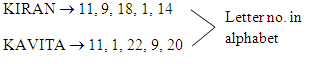Courses

# Coding Decoding - MCQ Test

## 10 Questions MCQ Test Quantitative Aptitude for Banking Preparation | Coding Decoding - MCQ Test

Description
This mock test of Coding Decoding - MCQ Test for UPSC helps you for every UPSC entrance exam. This contains 10 Multiple Choice Questions for UPSC Coding Decoding - MCQ Test (mcq) to study with solutions a complete question bank. The solved questions answers in this Coding Decoding - MCQ Test quiz give you a good mix of easy questions and tough questions. UPSC students definitely take this Coding Decoding - MCQ Test exercise for a better result in the exam. You can find other Coding Decoding - MCQ Test extra questions, long questions & short questions for UPSC on EduRev as well by searching above.
QUESTION: 1

### If in a certain code language 'SUMIT' is written as '82'. How will you code 'NISHA' in the same language?

Solution:

SUMIT → S + U + M + I + T → 19 + 21 + 13 + 9 + 20 ⇒ 82

Thus

NISHA → N + I + S + H + A → 14 + 9 + 19 + 8 + 1 ⇒ 51

QUESTION: 2

### If in a certain code language 'RAMAN' is written as '5' and 'PRASHANT' is written as '8'. How will you code 'TIMTIM' in the same language?

Solution:

Total no. of letters in given word.

QUESTION: 3

### If in a certain code language 'KIRAN' is written '11918114'. How will you code'KAVITA' in the same language?

Solution:QUESTION: 4

If in a certain code language 'CBE' is written as '6, 5, 8'. How will you code 'AMIT' in the same language?

Solution:

CBE → C + 3, B + 3, E + 3 → 6, 5, 8

AMIT → A + 3, M + 3, I + 3, T + 3 → 4, 16, 12, 23

QUESTION: 5

If in a certain code language 'ORANGE' is written as 'LIZMTV'. How will you code 'BANANA' in the same language?

Solution:

Opposite letters.

QUESTION: 6

If in a certain code language 'MADHU' is written as '3'. How will you code 'NISHA' in the same language?

Solution:

Sum of all consonant letters – Sum of all vowel letters

=> (N,S,H) - (I,A)

=> (14 + 19 + 8) - (9 + 1)

=> 41 - 10

=> 31

QUESTION: 7

If in a certain code language 'SET' is written as '1900'. How will you code 'ARM' in the same language?

Solution:

S × E × T → 19 × 5 × 20 = 1900

A × R × M → 1 × 18 × 13 = 234

QUESTION: 8

If in a certain code language 'SINGLE' is written as '994725'. How will you code 'DOUBLE' in the same language?

Solution:

SINGLE → 199, 147, 12, 5 → 994725

DOUBLE → 4, 15, 212, 125 → 451225

QUESTION: 9

If A = 1, SON = 48 then MART = ?

Solution:

Sum of all letters in given word.

QUESTION: 10

If in a certain code language 'SUPER' is written as 'RTTVOQDFQS'. How will you code 'TERROR' in the same language?

Solution:

Both nearest letters of the words letter.